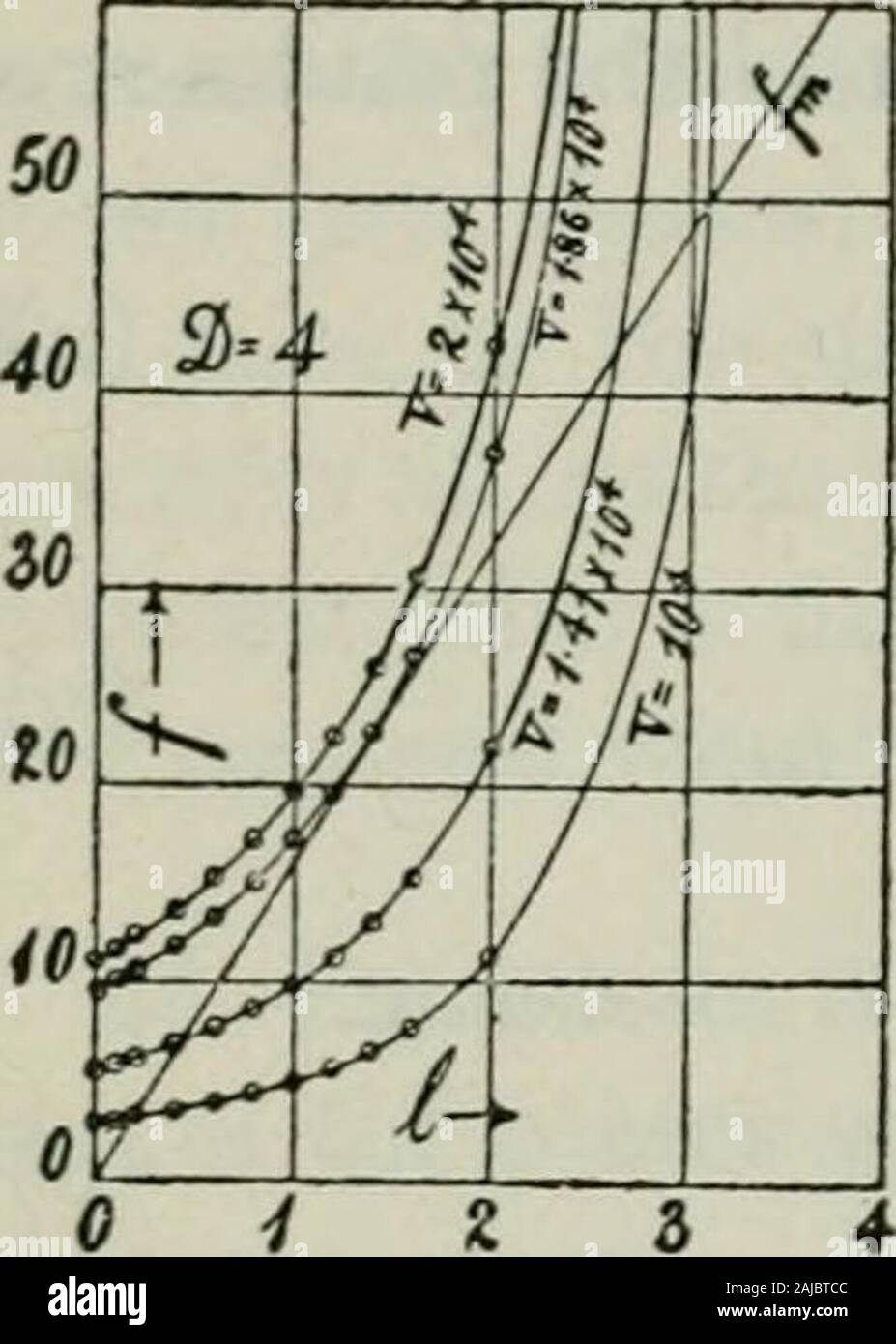# The diffusion of gases through liquids and allied experiments . 44 THB DIFFUSION OF GASES THROUGH To compute/j, a suitable value of D and V must be assumed. Let Z) = 4cm. and Kin succession 10^, io^Xi.41, io^Xi.86, 10^X2 volts. Since /,= FV2.262Xio«(4-/) the successive curvilinear lines of the diagram are obtained. The lowesthave two intersections each; the one corresponding to stability being at 5and the unstable one at us. For at s, a lowering of the disk means anexcessive upward electric force, while any rise of the disk means an excessivedownward and mechanical force. Just the reverse is t## Image details

Contributor:

The Reading Room / Alamy Stock Photo

Image ID:

2AJBTCC

File size:

Releases:

Model - no | Property - noDo I need a release?

Dimensions:

1335 x 1872 px | 22.6 x 31.7 cm | 8.9 x 12.5 inches | 150dpi

This image is a public domain image, which means either that copyright has expired in the image or the copyright holder has waived their copyright. Alamy charges you a fee for access to the high resolution copy of the image.

This image could have imperfections as it’s either historical or reportage.

The diffusion of gases through liquids and allied experiments . 44 THB DIFFUSION OF GASES THROUGH To compute/j, a suitable value of D and V must be assumed. Let Z) = 4cm. and Kin succession 10^, io^Xi.41, io^Xi.86, 10^X2 volts. Since /,= FV2.262Xio«(4-/) the successive curvilinear lines of the diagram are obtained. The lowesthave two intersections each; the one corresponding to stability being at 5and the unstable one at us. For at s, a lowering of the disk means anexcessive upward electric force, while any rise of the disk means an excessivedownward and mechanical force. Just the reverse is true at us. The twoupper curves have one contact and no contacts, respectively. Hence, whenF=io*Xi.86 and i? = 4, the disk will just be onthe point of rising without interruption. The maxi-mum rise compatible with stability would be 4/3cm. The rise for F= 10* and io*Xi.4i would beroughly 0.18 and 0.43 cm., respectively. To compute the potential of the maximum point ofstability for D = 3/= 4, equation (3) becomes 1/^ = 2.262X10*-^ Pg — Z)^ =1.86X10* volts.. R 27 The values of / for the stable positions of the disk incase of different values of D and V are not so easilyfound, in view of the cubic equation (3). When /is very small, however, i. e., for values of V lessthan 10* volts, / may be neglected in comparisonwith D and the equation becomes Fig. 14.—Chart showingthe forces actuatingdisk for different po-tential differences anddisplacements of disk. 7^ = 2.262X10^ R pgDH (9) Hence, if F=io^ the disk would only rise about 0.018 centimeter. ForF=io2 to 0.00018 cm., etc., so that even the interferometer could notindicate more than about 10 volts. If, however, D is also reduced, say to0.5 cm., or 8 times, the rise will be / = 0.0000092 cm. per volt; for D = o.icm., ^ = 0.00115 cm., etc. Hence with the use of the interferometer and small values of D, there isno reason why ultimately single volts should escape measurement exceptfor the capillary forces involved in flotation, whe

Save up to 70% with our image packs